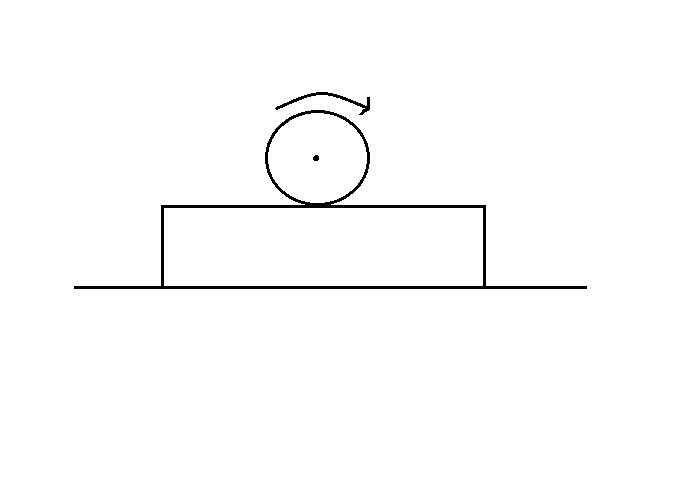# Distance moved till rolling.A horizontal plank having mass $m$ lies on a smooth horizontal surface. A sphere of same mass and radius $R$ is spined to an angular frequency $\omega$ and gently placed on the plank. If coefficient of friction between the plank and the sphere is $\mu$, find the distance moved by the plank till the sphere starts pure rolling on the plank. The plank is long enough. If answer is of the form $\frac { a }{ b } \frac { { { \omega }^{ 2 }R }^{ 2 } }{ \mu g }$, find $a+b$ where $a$ and $b$ are two co-primes integers.

×

Problem Loading...

Note Loading...

Set Loading...